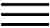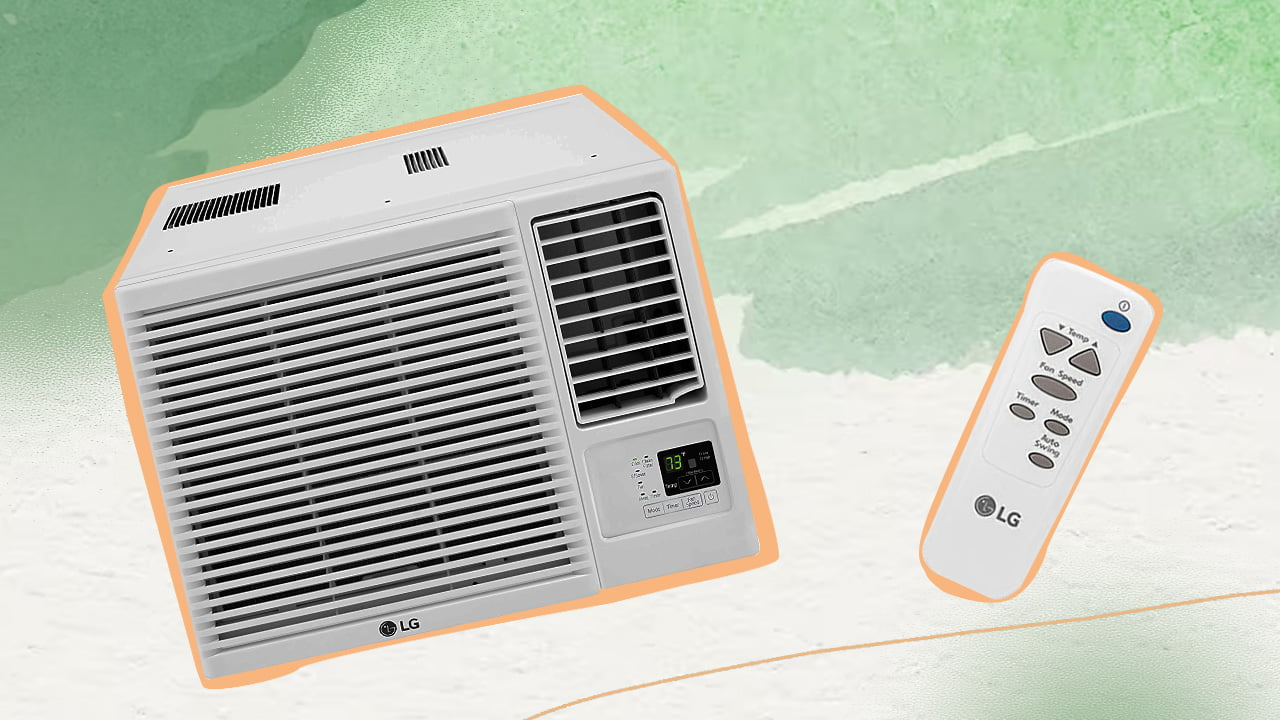X# BTU To kW Air Conditioning CalculatorBTU is a unit of heat commonly used in the US and UK to measure the cooling capacity of ACs. The rest of the world prefers using kW for the same, which is a unit of power by definition. Converting BTU to kW with the calculator provided here can help determine the different energy specifications of an AC.

## Convert BTU To kW Calculator

BTU To kW Air Conditioning Calculator

Switch

kW to BTU Air Conditioning Calculator

Switch

When it comes to heating, ventilation, and air conditioning (HVAC) systems, an air conditioner machine is the best means to control indoor air quality.

On that note, the heating and cooling capacity of such air conditioners is measured in units like BTU or kilowatts. Sometimes they are used interchangeably, which can become problematic for regular people to understand.

That is why I have included a handy BTU to kW calculator in this brief guide for your convenience. If you are curious about how these two units are related and what they imply, then keep reading!

## What Do BTU And kW Mean?Simply put, both of these units are used to measure the air conditioner capacity, as I have already mentioned before. To explain it a bit more technically, I’ll need to delve into the basic science of heating and cooling systems.

BTU stands for British thermal units, and it is used for the measurement of heat. By definition, it is the quantity of heat energy necessary to increase the temperature of a pound (mass) of water by one degree Fahrenheit. It is mostly used as a basic unit of measurement in the United States and the United Kingdom.

On the other hand, a kilowatt is a unit of power (physics), and it is used to measure the amount of energy consumed by an appliance in a given time. In the case of HVAC systems, the kilowatt can be used to indirectly measure the amount of heat given out by the machine.

Do not be confused by these two statements, for they both imply the same thing. The higher the energy consumption of an appliance, the more heat it will generate, and vice versa.

Kilowatt is the more widely used unit in other parts of Europe and the rest of the world. Here, I should mention that a kilowatt is actually a large unit that is derived from a watt. This is the standard recommended unit of power according to the International System of Units (otherwise known as the SI system). In this regard, one kilowatt is equal to 1,000 watts.

## What Is The Relation Between BTU And kW?

Technically speaking, the BTU is not exactly the same as kW, as you can already tell from the above discussion. The BTU is a unit that is used to measure energy, while the kW is a unit of power. Keeping that in mind, the actual equivalent unit to kW is BTU/hr, which is the heating or cooling provided by a split or window air conditioner in an hour.

On a similar note, the unit that is equivalent to BTU is a kilowatt-hour or kWh, which measures the total energy consumption of your air conditioning equipment. The metric equivalent of BTU is the joule, which is the SI unit of energy.

### Converting The Units

Now that you know the distinction between these units, it is time to address the relationship between them, which will help with the conversion. Both the units of BTU and BTU/hr are smaller compared to their respective counterparts.

In that context, one British thermal unit is roughly equivalent to 0.00029307107 kWh. Thus, if you wish to convert BTU to kW, you need to multiply the AC’s BTU rating by the above number. And if you want to convert from kW to BTU, you need to divide the kW rating by the above figure.

Likewise, if you are looking for the BTU/hr to kW conversion, you can do it in one simple step. Simply divide the BTU/hr rating of the AC by 3412.14, and you will get the corresponding kW rating. Conversely, by multiplying the kW figure of your AC by the above number, you will get the BTU/hr rating of your AC.

Or, you can use an online conversion calculator to avoid the hassle of manual calculations. In fact, you can use these calculators to convert different aspects of your AC. For instance, if you use a watt to amps calculator, you can determine the electrical current used by your AC.

Similarly, with a suitable calculator to convert between the COP and EER numbers, you can determine the performance of your system in terms of electricity consumption. This can be particularly helpful in calculating monthly energy spending. You can even determine how many BTUs you need for a room using a BTU calculator.

Tip

Most manufacturers today provide a BTU to kW chart (and other relevant conversion charts) to help with this aspect. So, you should always consult them before making the purchase.## Final Words

That brings me to the end of this brief guide. You will find the information to be helpful if you are doing some energy calculations for your AC or are simply curious.

It is always a wise idea to determine these aspects before you purchase the air conditioner. That way, you will have good control over your usage habits and reduce your monthly electricity bills. Or you could impart the knowledge to others and help them out with their purchase.

Anyway, now it is time for me to say goodbye. See you around, folks!